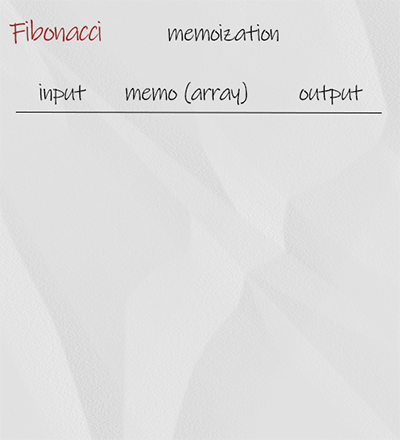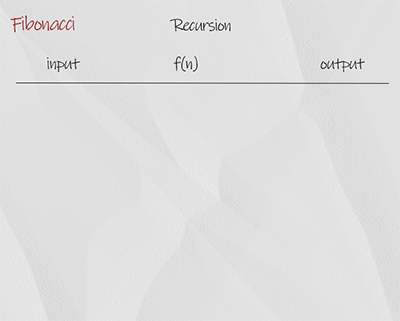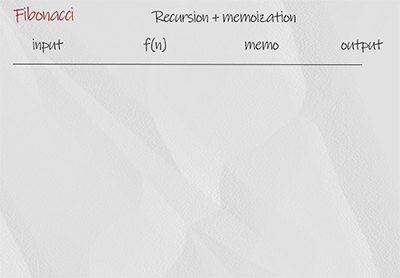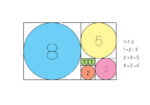In this post, we are going to find n-th Fibonacci number for any given number n. There are 4 implementations. They are iteration, dynamic programming, recursion and memoization.

### Iterative solution

We use for loop starting from 2. In each iteration, variable sum in the sum of previous two number a and b. When finish, return sum. The time complexity is O(n), space complexity is O(1). It is the best solution.

## Doodle### Dynamic programming

The basic idea is the same as iteration. In additional, we use an array to save all the result. At the end we return the value with the index of the input number from the array. The time complexity is O(n), space complexity is O(n).

## Doodle### Recursive solution

The recursion is a simple implementation of the concept, in which each number is the sum of the previous of two number. The base condition is when input is 0 or 1, it return the number itself. It is not efficient. For example, when the input is 4, it calls f(2) twice. So there is overlapping. The time complexity is O(2^n), space complexity is O(n). It is the worst solution.

## Doodle### Memoization

This method is to overcome the overlapping in recursion using memoization. The solution uses an array to store the partial result. For example, if the result of f(2) is saved, next time the function finds the result in array and returns it. Memoization is one of techniques in called dynamic programming. The time complexity is O(n), space complexity is O(n).

## Doodle